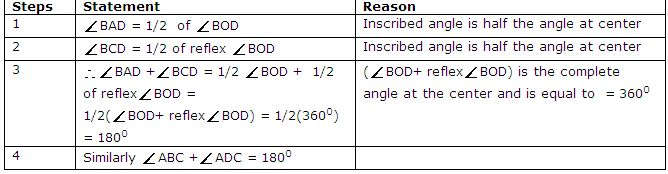Name: ___________________Date:___________________

 Email us to get an instant 20% discount on highly effective K-12 Math & English kwizNET Programs!

### High School Mathematics - 28.3 Cyclic Quadrilateral

 Cyclic Quadrilateral: A quadrilateral whose vertices lie on the circumference of a circle is called cyclic quadrilateral. Theorem: Opposite angles of a cyclic quadrilateral are supplementary.Proof:Directions: Solve the following.Name: ___________________Date:___________________

### High School Mathematics - 28.3 Cyclic Quadrilateral

 Q 1: Answer: Q 2: PQRS is a cyclic quadrilateral, if angleQ = angleR = 65o, find angle P and angle S.115o120o100o Q 3: Answer: Q 4: PQRS is a cyclic quadrilateral whose diagonals intersect at A.If ang SQR = 80o, angle QPR = 30o, find angle SRQ.90o70o80o Q 5: Answer: Q 6: Prove that when a parallelogram is inscribed in a circle it becomes a rectangleAnswer: Q 7: A pair of opposite sides of a cyclic quadrilateral is equal. Prove that their diagonals are equal.Answer: Q 8: Prove that the exterior angle of a cyclic quadrilateral is equal to its interior opposite angle.Answer: Question 9: This question is available to subscribers only! Question 10: This question is available to subscribers only!